# Spectral operator

spectral measure

A bounded linear operatormapping a Banach spaceinto itself and such that for the-algebra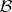of Borel subsetsin the plane there is a resolution of the identitywith the following properties: 1) for any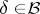the projector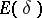reduces, that is,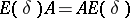and the spectrum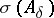lies in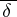, whereis the restriction ofto the invariant subspace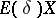; 2) the mapping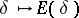is a homeomorphism of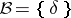into the Boolean algebra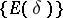; 3) all projectorsare bounded, that is,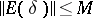,; and 4) the resolution of the identityis countably additive in the strong topology of, that is, for anyand any sequence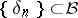of pairwise disjoint sets,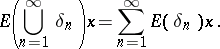The concept of a spectral operator can be generalized to the case of closed unbounded operators. In 1), the additional requirement is then that the inclusion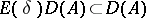holds, where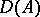is the domain of definition of, and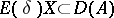for bounded.

All linear operators on a finite-dimensional space and all self-adjoint and normal operators on a Hilbert space are spectral operators. For example, the operator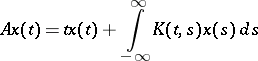on,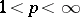, is spectral on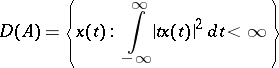if the kernelis the Fourier transform of a Borel measureon the plane of total variation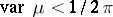and is such that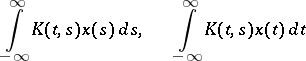are bounded linear operators on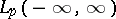.

Spectral operators have many important properties, such as: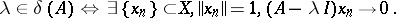Ifis separable, the point and residual spectra ofare at most countable.